MATLAB tutorial

•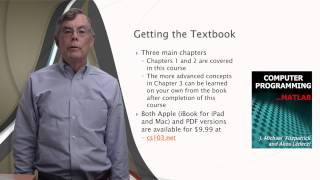WatchLesson 1: 1. Introduction (Old version)
•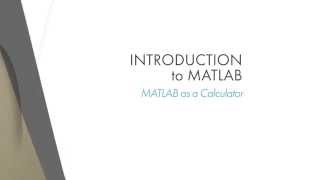WatchLesson 1.4: MATLAB as a Calculator
•WatchLesson 1.5: Syntax and Semantics
•WatchLesson 1.6:The MATLAB Help System
•WatchLesson 1.7: Introduction to Plotting in MATLAB
•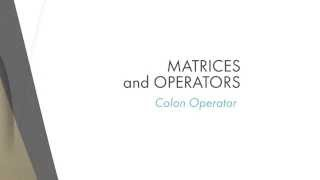WatchLesson 2.2: The Colon Operator
•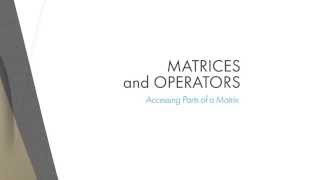WatchLesson 2.3: Accessing Parts of Matrix
•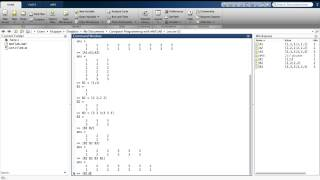WatchLesson 2.4 Combining and Transforming Matrices
•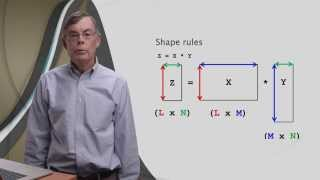WatchLesson 2.5: Arithmetic Part 1 (Old Version)
•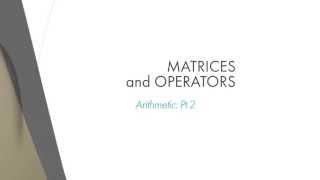WatchLesson 2.6: Arithmetic Part 2
•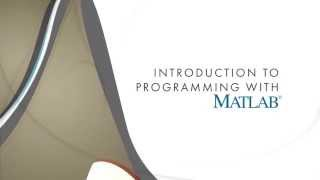WatchLesson 2.7: Operator Precedence
•WatchLesson 3.1: Introduction to Functions
•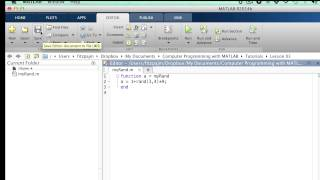WatchLesson 3.2: Function Input/Output
•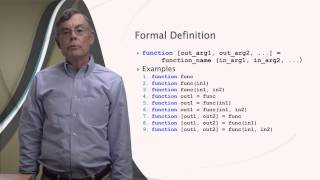WatchLesson 3.3: Formal Definition of Functions
•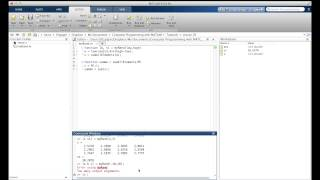WatchLesson 3.4: Sub-functions
•WatchLesson 3.5: Scope
•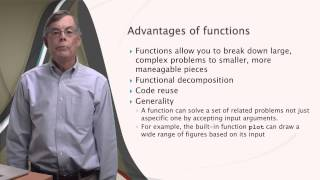WatchLesson 3.6: Advantages of Functions
•WatchLesson 3.7: Scripts
•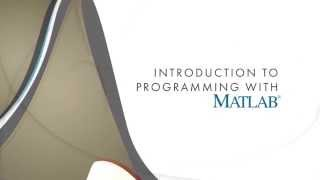WatchLesson 4.1: Introduction to the Programmer's Toolbox
•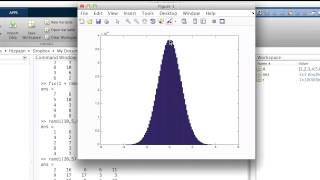WatchLesson 4.2: Matrix Building
•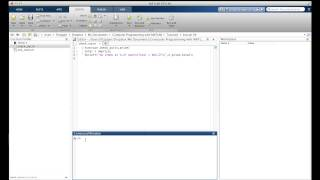WatchLesson 4.3: Input/Output
•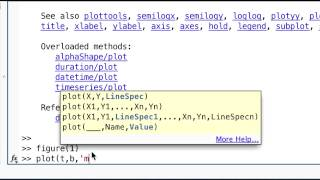WatchLesson 4.4: Plotting
•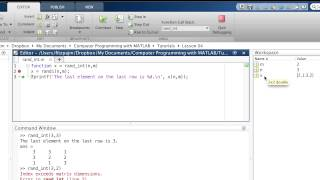WatchLesson 4.5: Debugging
•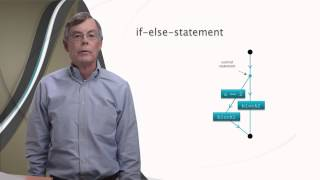WatchLesson 5.1: Selection (Branching) in MATLAB
•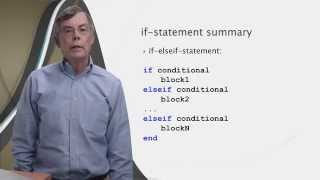WatchLesson 5.2: If-Statement cont'd.
•WatchLesson 5.3: Relational and Logical Operators
•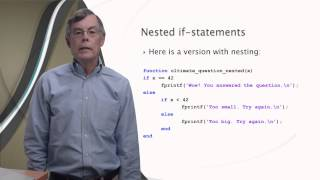WatchLesson 5.4: Nested If-statements
•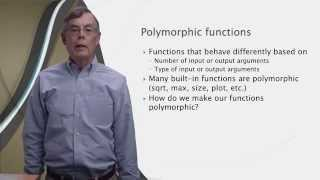WatchLesson 5.5: Variable Number of Arguments
•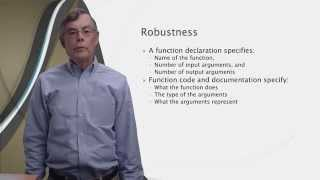WatchLesson 5.6: Robustness
•WatchLesson 5.7: Persistent Variables
•WatchLesson 6.1 for-loops in MATLAB
•WatchLesson 6.2 while-loops in MATLAB
•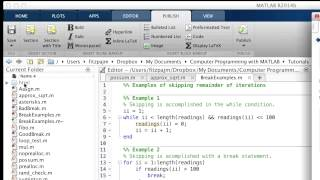WatchLesson 6.3: Break-statement in MATLAB
•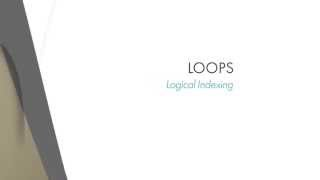WatchLesson 6.4: Logical indexing in MATLAB
•WatchLesson 6.5: Preallocation
•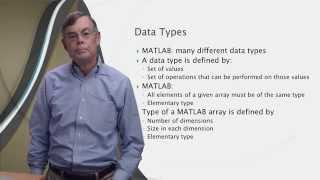WatchLesson 7.1: Introduction to data types
•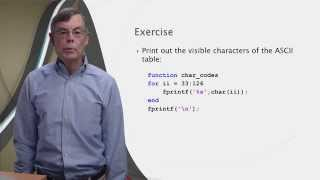WatchLesson 7.2: Strings (old version)
•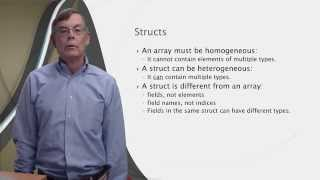WatchLesson 7.3 Structs
•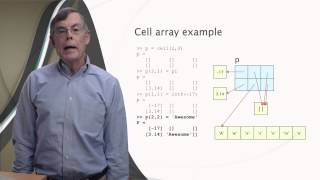WatchLesson 7.4: Cells
•WatchLesson 8.1: Introduction to files in MATLAB
•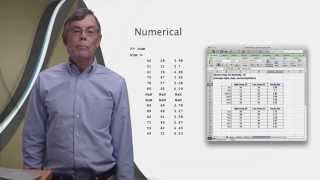WatchLesson 8.2: Excel files in MATLAB
•WatchLesson 8.3: Text Files

Learn to Code is an online, interactive tutorial that teaches the basics of programming using MATLAB. Let’s get started. In the tutorial, students will solve the problem of finding the closest meet-up location on a map by creating an algorithm. They’ll learn how to work with variables, functions, and vectors. No prior …